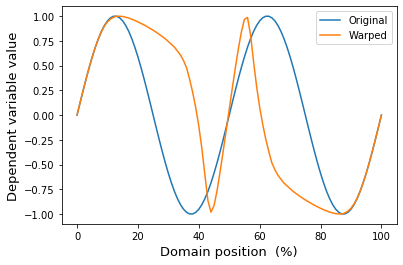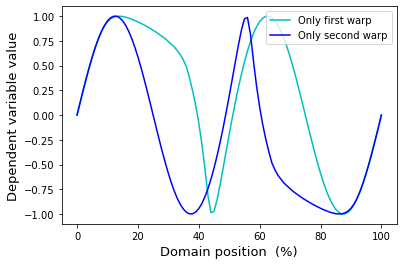# Scripting sequential manual warps¶

The mwarp1d GUI supports sequential manual warps for arbitrarily fine control over local warping effects. This control is replicated in the SequentialManualWarp class, as demonstrated below.

In :

%matplotlib inline

In :

import numpy as np
from matplotlib import pyplot as plt
import mwarp1d

#define first warp:
Q     = 101                      #domain size
warp0 = mwarp1d.ManualWarp1D(Q)  #constrained Gaussian kernel warp object
warp0.set_center(0.10)           #relative warp center (0 to 1)
warp0.set_amp(0.3)               #relative warp amplitude (-1 to 1)
warp0.set_tail(0.0)              #relative warp tail (0 to 1)

#define second warp:
warp1 = mwarp1d.ManualWarp1D(Q)
warp1.set_center(0.90)
warp1.set_amp(-0.3)
warp1.set_tail(0.0)

#create and apply sequential warps
seq   = mwarp1d.SequentialManualWarp()
seq.append( warp0 )
seq.append( warp1 )
y     = np.sin( np.linspace(0, 4*np.pi, Q) )  #an arbitary 1D observation
yw    = seq.apply_warp_sequence(y)            #sequentially warped 1D observation

#plot:
plt.figure()
ax = plt.axes()
ax.plot(y, label='Original')
ax.plot(yw, label='Warped')
ax.legend()
ax.set_xlabel('Domain position  (%)', size=13)
ax.set_ylabel('Dependent variable value', size=13)
plt.show()Individual warps can be accessed and applied individually like this:

In :

w0    = seq.warps
w1    = seq.warps

yw0   = w0.apply_warp(y)
yw1   = w1.apply_warp(y)
yw01  = w1.apply_warp(yw0)

#plot:
plt.figure()
ax = plt.axes()
ax.plot(yw0,  'c', label='Only first warp')
ax.plot(yw1,  'b', label='Only second warp')
# ax.plot(yw01, 'orange', label='Sequentially warped')
ax.legend()
ax.set_xlabel('Domain position  (%)', size=13)
ax.set_ylabel('Dependent variable value', size=13)
plt.show()The main value of the SequentialManualWarp class is to apply manual warps (that were constructed in the GUI) to other data. For example, if the original data represent the time series of a three-component force vector, and if the warps were created using only the X component time series, then the SequentialManualWarp class can be used to apply those warps to the Y and Z components. This is demonstrated in the “Applying GUI warps” example.